Groups of (grouping)
Arrays
Number Lines
100

Which multiplication equation is being represented? Write the factors and product.4 x 2 = 8

100

Which multiplication equation is being represented? Write the equation and product.

5 + 5 + 5 + 5

4 x 5 = 20

100

Which multiplication equation is being represented? Write the factors and product.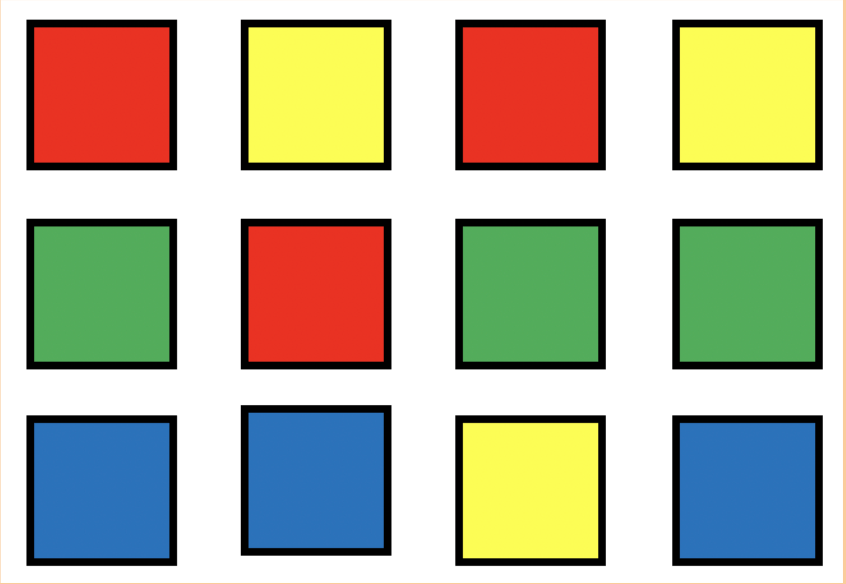3 x 4 = 12

100

Which multiplication equation is being represented on the number line? Write the factors and the product.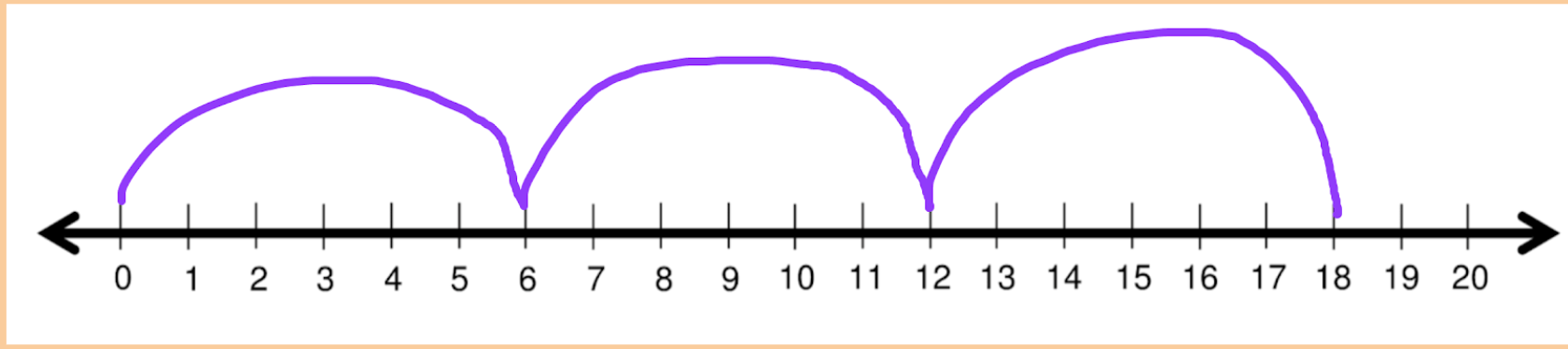3 x 6 = 18

200

Using the grouping (groups of) method, draw out 5x3 and write the product.

200

Using the repeated addition method, write out 3 x 4 and the product.

4 + 4 + 4 = 12

200

Draw out 2 x 8 using the arrays model and write the product.

200

Which multiplication equation is being represented on the number line? Write the factors and the product.5 x 2 = 10

300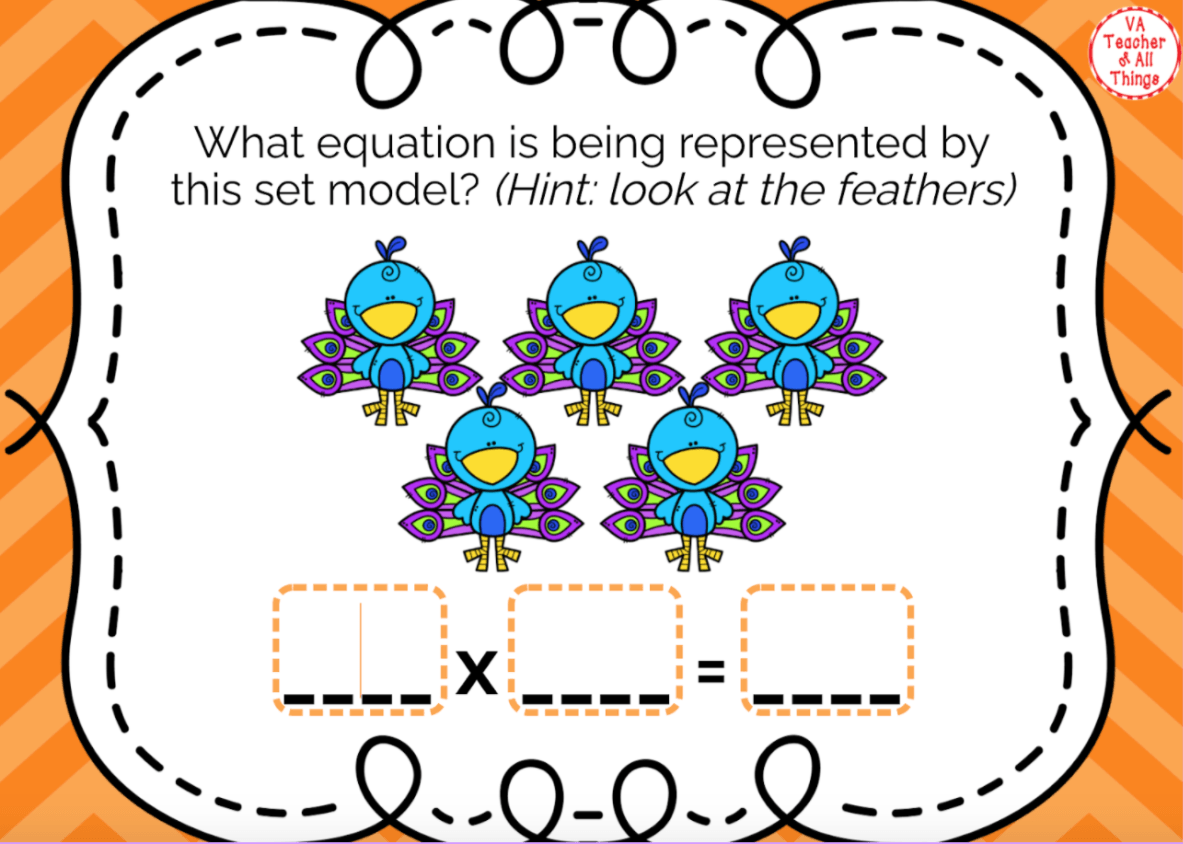5 x 6 = 30

300

Which multiplication equation is being represented? Write the equation and product.

11 + 11 + 11 + 11 + 11

5 x 11 = 55

300

Which multiplication equation is being represented? Write the equation and product.5 x 7 = 35

300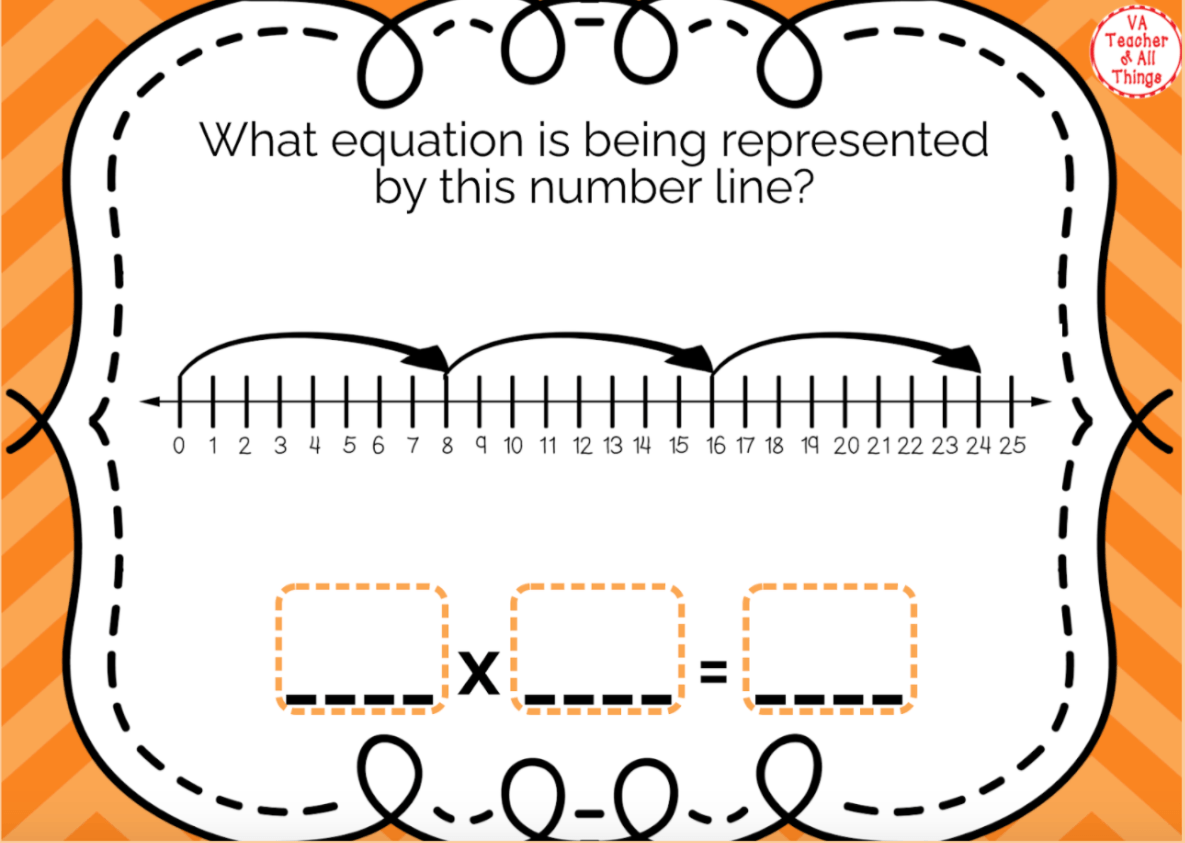3 x 8 = 24

400

Using the grouping (groups of) method, draw out 3x9 and write the product.

400

Using the repeated addition method, write out 6 x 8 and the product.

8 + 8 + 8 + 8 + 8 + 8 = 48

400

Draw out 6 x 7 using the arrays model and write the product.

400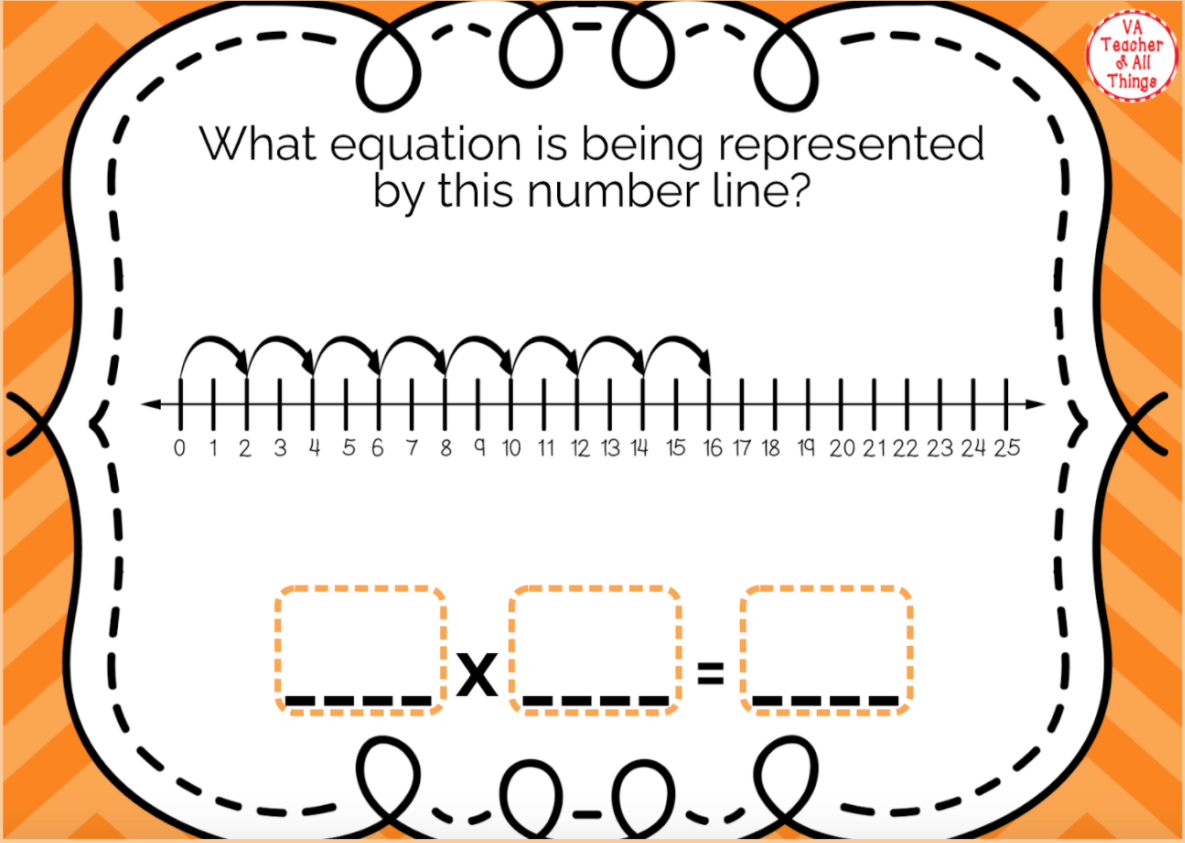8 x 2 = 16

Click to zoom